Next: Change of Pivot Up: General Discussions without Momentum Previous: Helix Parametrization

# Generalities of Track Fitting

Track fitting is the procedure to determine helix parameters by fitting a set of coordinates measured in a tracking detector to a helix. The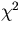to minimize has, in general, the following form: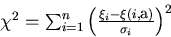(3)
where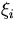is i-th measured coordinate,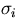is its error, and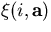is the expected i-th coordinate, when the helix parameter vector is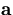. What we need is the helix parameter vector which zeros the first derivative of: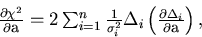(4)
where we have defined the i-th residual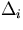by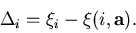(5)
This can be numerically found by iteratively from the following equation (multi-dimensional Newton's method):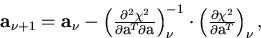(6)
where the left-hand side is the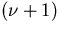-th estimate of the helix parameter vector based on the knowledge on the right-hand side of its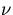-th estimate together with the first and the second derivatives of thethereat. The second derivative is given by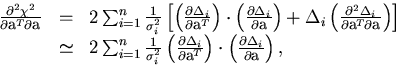(7)
where in the last line we have deliberately left out the second derivatives of's in order to make positive definite the second derivative matrix of. When the initial estimate of the parameter vector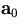is a good approximation, the parameter vector converges rapidly after a few times of iterations. Nevertheless, it is usually recommended to multiply the diagonal elements of the second derivative matrix by some constant which is greater than unity and try again, when theincreased for the new estimate of the parameter vector. This prescription renders the parameter change vector along the opposite gradient direction in such cases and stabilizes the fit.

When the fit converges, the error matrix for the parameter vector is obtained to be(8)

It should be emphasized that we have to carefully choose the helix parametrization so as to numerically stabilize the fitting procedure: the helix parameters should stay small and continuously change during the fit. Notice that our parametrization allows a continuous transition from a negative charge solution to a positive charge solution: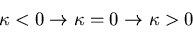with other parameters stay the same. Since this transition implies that the center of the helix jumps from a point infinitely away on one side of the track to another infinitely away point on the other side of the track, it changes the meaning of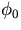discretely by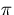.Next: Change of Pivot Up: General Discussions without Momentum Previous: Helix Parametrization
Keisuke Fujii
12/4/1998